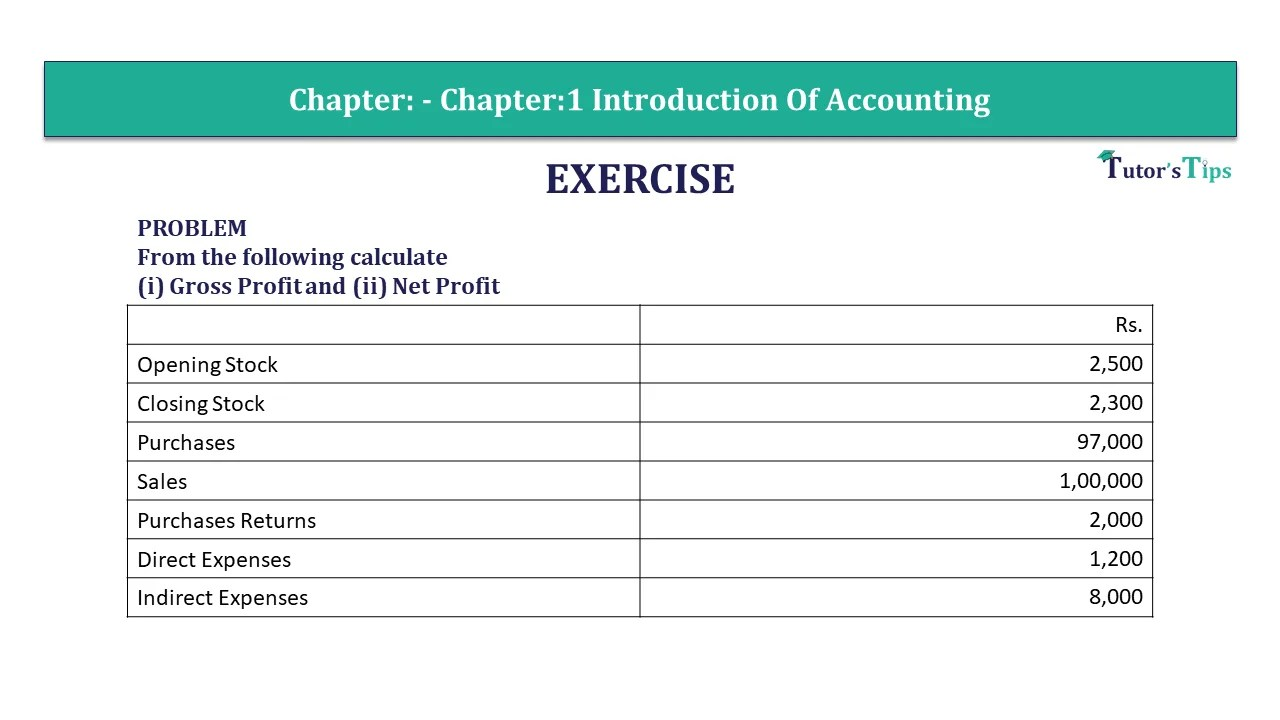# Problem 4 Chapter 1 – Unimax Publications of Class 11Problem 4- CH-1 Solution-min

Problem 4 Chapter 1 – Unimax

PROBLEM
From the following calculate
(i) Gross Profit and (ii) Net Profit

 Rs. Opening Stock 2,500 Closing Stock 2,300 Purchases 97,000 Sales 1,00,000 Purchases Returns 2,000 Direct Expenses 1,200 Indirect Expenses 8,000

## The solution of Problem 4 Chapter 1 – Unimax

 (i) Cost of goods sold = Opening Stock + Net Purchases + Direct Expenses – Closing Stock Net Purchases = Purchases-Purchases Returns = Rs. 97,000-2,000 = Rs. 95,000 Cost of goods sold = Rs. 2,500+95,000+1,200-2,300 = Rs. 96,400
 (ii) Gross loss = Net Sales – Cost of goods sold = Rs. (1,00,000-96,400) = Rs. 3,600
 (iii) Net Profit = Gross Profit-Indirect Expenses (Or) Net Loss = Indirect Expenses-Gross Profit = Rs. 8,000-3,600 = Rs. 4,400

Note: Since, Indirect Expenses are more than gross profit. So, the result is net loss.

This is all about the Problem 4 Chapter 1 – Unimax. You can check out the following article to better understand:

Financial Accounting Terminology

You Can also read all above articles in Hindi on our Hindi Website

Financial Accounting Terminology – Explained in Hindi

Comment if you have any doubt in the Problem 4 Chapter 1 – Unimax.

You can also Check out the solved question of other Chapters: –

## Advanced Accountancy – Unimax Class 11 – 2021 – Solution.

Part-I

• Chapter No. 1 – Introduction of Accounting
• Chapter No. 2 – Theory Base of Accounting
• Chapter No. 3 – Vouchers and Transactions
• Chapter No. 4 – Journal
• Chapter No. 5 – Goods and Services Tax (GST) : An Introduction
• Chapter No. 6 – Ledger
• Chapter No. 7 – Special Purpose Book – Cash Book
• Chapter No. 8 – Other Subsidiary Books
• Chapter No. 9 – Trial Balance
• Chapter No. 10 – Rectification of Errors
• Chapter No. 11 – Depreciation
• Chapter No. 12 – Provision and Reserves
• Chapter No. 13 – Bank Reconlciliation Statement
• Chapter No. 14 – Bills of Exchange

Students may Choose only one part from the Part II and Part III

Part-II

• Chapter No. 15 – Financial Statements (Without Adjustments)
• Chapter No. 16 – Financial Statements (With Adjustments)
• Chapter No. 17 – Accounts from Incomplete Records – Single Entry System

Part-III

• Chapter No. 18 – Introduction to Compurters and Accounting information System
• Chapter No. 19 – Computerised Accounting
• Chapter No. 20 – Accounting Software : Tally
• Chapter No. 21 – Data Base System
• Chapter No. 22 – Concept of Entity and Relationship

You can also Check out the other Books’ Solution: –

error: Content is protected !!